The CATMOD Procedure
 Computational Method

The notation used in PROC CATMOD differs slightly from that used in the literature. The following table provides a summary of the basic dimensions and the notation for a contingency table. See the section Computational Formulas for a complete description.

### Summary of Basic Dimensions= number of populations or samples (= number of rows in the underlying contingency table)= number of response categories (= number of columns in the underlying contingency table)= number of response functions computed for each population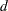= number of parameters

### NotationDenotes a column vector of 1s.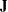Denotes a square matrix of 1s.Denotes the sum over all the possible values of.Denotes the row sum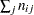.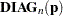Denotes the diagonal matrix formed from the firstelements of the vector.Denotes the inverse of.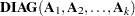Denotes a block diagonal matrix with thematrices on the main diagonal.

Input data can be represented by a contingency table, as shown in Table 28.5.

Table 28.5 Input Data Represented by a Contingency Table

Response

Population

1

2Total

1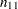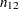2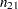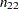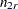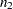s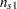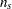Previous Page | Next Page | Top of Page